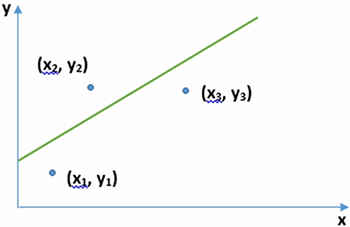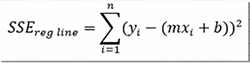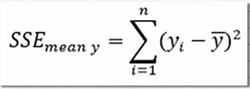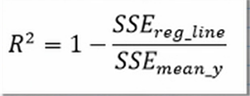# Coefficient of Determination: Definition, Formula & Example

Lesson Transcript
Instructor: Artem Cheprasov

Artem has a doctor of veterinary medicine degree.

The coefficient of determination is an important quantity obtained from regression analysis. In this lesson, we will show how this quantity is derived from linear regression analysis, and subsequently demonstrate how to compute it in an example. Updated: 01/15/2020

## Pizza!

Do you have a favorite pizza place? Let's just suppose you want to find out how additional pizza toppings affect the total cost of a pizza across all the different pizza places in your city. To do this you pick up the phone and start calling all the different pizza places, writing down the total cost of the pizza with one, two, three, etc., toppings on it at each place.

Once you are done, you will need to fit your data with an equation and, just as importantly, find out if your mathematical model for the data is a good fit.

An error occurred trying to load this video.

Try refreshing the page, or contact customer support.

Coming up next: Pearson Correlation Coefficient: Formula, Example & Significance

### You're on a roll. Keep up the good work!

Replay
Your next lesson will play in 10 seconds
• 0:03 Pizza!
• 0:38 Coefficient of…
• 3:07 Coefficient of…
• 4:17 Lesson Summary
Save Save

Want to watch this again later?

Log in or sign up to add this lesson to a Custom Course.

Timeline
Autoplay
Autoplay
Speed Speed

## Coefficient of Determination Derived

In this lesson, we will talk about a statistical construct that is used to estimate the predictive power of you model. The coefficient of determination denoted as big R2 or little r2 is a quantity that indicates how well a statistical model fits a data set. In mathematical terms, it specifies how much of the variation in the dependent variable y is characterized by a variation in the independent variable x.

You may be wondering what r is, since we only defined r2. You can think of the correlation coefficient denoted as big R or little r as a measure of the statistical relationship between x and y. As the focus of this lesson is the coefficient of determination, just remember that r stands for the correlation coefficient, simple as that.Okay, let's do a simple derivation of the coefficient of determination. In the image, you see we start with plot containing a set of points, x and y, in which we assume there is a linear relationship between the x and y variables. Note that this linearity assumption is made to simplify the derivation and that a similar process can be used for non-linear models.

Shown is a plot with three sample points. We now try to find the regression line, which a line of best fit for the data points. The line in green shows one attempted line of best fit.

We can simplify this line by the equation y = mx + b, which is the standard equation for a line. To calculate the sum of the squared errors between each data point and our line of best fit, we perform the follow computation:In this equation the term SSEreg line stands for the square sum of errors from the regression line.

Our next step is to find out how the y value of each data point differs from the mean y value of all the data points. In particular we need to compute the sum of the squares of these differences to the right of the equals sign, as shown below.The term SSEmean y line stands for squared sum of errors from the mean y value.

We now have everything we need to compute the coefficient of determination, as you can see below.To unlock this lesson you must be a Study.com Member.
Create your account

### Register to view this lesson

Are you a student or a teacher?

### Unlock Your Education

#### See for yourself why 30 million people use Study.com

##### Become a Study.com member and start learning now.
Back
What teachers are saying about Study.com
Create an account to start this course today
Used by over 30 million students worldwide